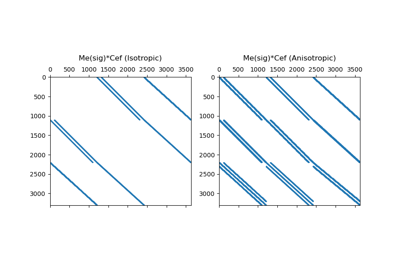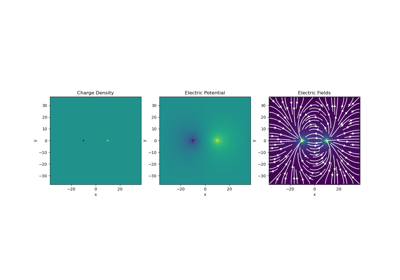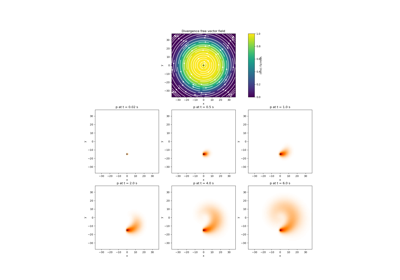# discretize.utils.sdiag#

discretize.utils.sdiag(v)[source]#

Generate sparse diagonal matrix from a vector.

This function creates a sparse diagonal matrix whose diagonal elements are defined by the input vector v. For a vector of length n, the output matrix has shape (n,n).

Parameters
v(n) numpy.ndarray or discretize.utils.Zero

The vector defining the diagonal elements of the sparse matrix being constructed

Returns
(n, n) scipy.sparse.csr_matrix or discretize.utils.Zero

The sparse diagonal matrix.

Examples

Use a 1D array of values to construct a sparse diagonal matrix.

>>> from discretize.utils import sdiag
>>> import numpy as np
>>> v = np.array([6., 3., 1., 8., 0., 5.])
>>> M = sdiag(v)


## Galleries and Tutorials using discretize.utils.sdiag#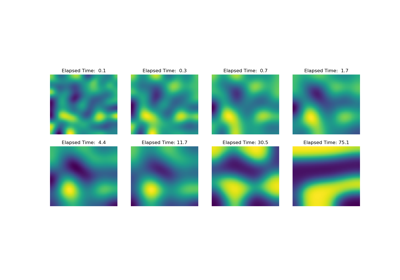Operators: Cahn Hilliard

Operators: Cahn Hilliard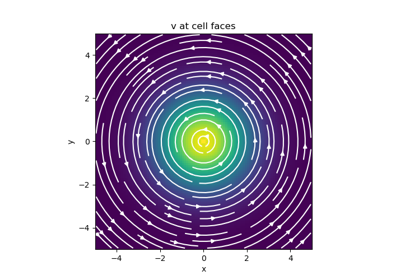Basic Inner Products

Basic Inner Products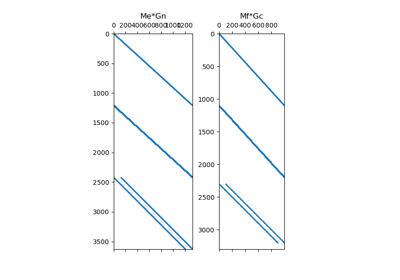Differential Operators

Differential Operators